UPSC  >  Number Systems - Finding Remainders, Number Theory

# Number Systems - Finding Remainders, Number Theory Notes | Study UPSC Prelims Paper 2 CSAT - Quant, Verbal & Decision Making - UPSC

## Document Description: Number Systems - Finding Remainders, Number Theory for UPSC 2022 is part of UPSC Prelims Paper 2 CSAT - Quant, Verbal & Decision Making preparation. The notes and questions for Number Systems - Finding Remainders, Number Theory have been prepared according to the UPSC exam syllabus. Information about Number Systems - Finding Remainders, Number Theory covers topics like Finding Remainders of a Product&nbsp;, (Derivative of Remainder Theorem), Concept of Negative Remainder, Finding Remainders Of Powers With The Help Of Remainder Theorem, Application Of Binomial Theorem In Finding Remainders, Wilson's Theorem and Number Systems - Finding Remainders, Number Theory Example, for UPSC 2022 Exam. Find important definitions, questions, notes, meanings, examples, exercises and tests below for Number Systems - Finding Remainders, Number Theory.

Introduction of Number Systems - Finding Remainders, Number Theory in English is available as part of our UPSC Prelims Paper 2 CSAT - Quant, Verbal & Decision Making for UPSC & Number Systems - Finding Remainders, Number Theory in Hindi for UPSC Prelims Paper 2 CSAT - Quant, Verbal & Decision Making course. Download more important topics related with notes, lectures and mock test series for UPSC Exam by signing up for free. UPSC: Number Systems - Finding Remainders, Number Theory Notes | Study UPSC Prelims Paper 2 CSAT - Quant, Verbal & Decision Making - UPSC
 Table of contents Finding Remainders of a Product (Derivative of Remainder Theorem) Concept of Negative Remainder Finding Remainders Of Powers With The Help Of Remainder Theorem Application Of Binomial Theorem In Finding Remainders Wilson's Theorem
 1 Crore+ students have signed up on EduRev. Have you?

Finding Remainders of a Product

(Derivative of Remainder Theorem)

(i) If ‘a1’ is divided by ‘n’, the remainder is ‘r1’ and if ‘a2’ is divided by ‘n’, the remainder is r2. Then,

If a1+a2 is divided by n, the remainder will be r1 + r2.
If a1 - a2 is divided by n, the remainder will be r1 - r2.
If a1 × a2 is divided by n, the remainder will be r1 × r2.

Concept of Negative Remainder

By definition, remainder cannot be negative. But in certain cases, you can assume that for your convenience. But a negative remainder in real sense means that you need to add the divisor in the negative remainder to find the real remainder.

Example:

If 21 is divided by 5, the remainder is 1 and if 12 is divided by 5, the remainder is 2. Then, if (21 + 12 = 33) is divided by 5, the remainder will be (1 + 2 = 3).
If (21 - 12 = 9) is divided by 5, the remainder will be 1 - 2 = - 1.
But if the divisor is 5, - 1 is nothing but 4 (9 = 5 × 1 + 4)
So, if 9 is divided by 5, the remainder is 4 and 9 can be written as 9 = 5 × 2 - 1.
So here - 1 is the remainder. So - 1 is equivalent to 4 if the divisor is 5. Similarly - 2 is equivalent to 3.
If (21 × 12 = 252) is divisible by 5, the remainder will be (1 × 2 = 2).

(ii) If two numbers ‘a1’ and ‘a2‘ are exactly divisible by n. Then their sum, difference and product is also exactly divisible by n.
i.e., If ‘a1’ and ‘a2’ are divisible by n, then

a1 + a2 is also divisible by n
a1 - a2 is also divisible by n
a1 × a2 is also divisible by n.

Example: 12 is divisible by 3 and 21 is also divisible by 3
Sol. So, 12 + 21 = 33, 12 - 21 = - 9 and 12 × 21 = 252 all are divisible by 3.

Finding Remainders Of Powers With The Help Of Remainder Theorem

Example 1: What is the remainder if 725 is divided by 6?
Solution: If 7 is divided by 6, the remainder is 1. So if 725 is divided by 6, the remainder is 1 (because 725 = 7 × 7 × 7… 25 times. So remainder = 1 × 1 × 1…. 25 times = 125).

Example 2: What is the remainder, if 363 is divided by 14.
Solution: If 33 is divided by 14, the remainder is - 1. So 363 can be written as (33)21.
So the remainder is (- 1)21 = - 1.
If the divisor is 14, the remainder - 1 means 13. (14 - 1 = 13) by pattern method.

Question for Number Systems - Finding Remainders, Number Theory
Try yourself:Find the remainder when 433 is divided by 7.

Application Of Binomial Theorem In Finding Remainders

The binomial expansion of any expression of the form
(a + b)n = nCo an + nC1 an-1 × b1 + nC2 × an-2 × b2 ..... + nCn-1 × a1 × bn-1 + nCn × bn
Where nCo, nC1, nC2, .... are all called the binomial coefficients
In general, nCr = n!/r!(n - r)!

There are some fundamental conclusions that are helpful if remembered:
(a) There are (n + 1) terms.
(b) The first term of the expansion has only a.
(c) The last term of the expansion has only b.
(d) All the other (n - 1) terms contain both a and b.
(e) If (a + b)n is divided by a, then the remainder will be bn such that bn < a.

Example 1: What is the remainder if 725 is divided by 6?
Solution.
(7)25 can be written (6 + 1)25. So, in the binomial expansion, all the first 25 terms will have 6 in it. The 26th term is (1)25. Hence, the expansion can be written 6x + 1. 6x denotes the sum of all the first 25 terms.

Since each of them is divisible by 6, their sum is also divisible by 6, and therefore, can be written 6x, where x is any natural number. So, 6x + 1 when divided by 6 leaves the remainder 1.

(OR)

When 7 divided by 6, the remainder is 1. So when 725 is divided by 6, the remainder will be 125 = 1.

Question for Number Systems - Finding Remainders, Number Theory
Try yourself:Remainder when 2510 is divided by 576?

Wilson's Theorem

If n is a prime number, (n - 1)! + 1 is divisible by n.
Lets take n = 5
Then (n - 1)! + 1 = 4! + 1 = 24 + 1 = 25 which is divisible by 5.
Similarly If n = 7
(n - 1)! + 1 = 6! + 1 = 720 + 1 = 721 which is divisible by 7.

Corollary
If (2p + 1) is a prime number (p!)2 + (- 1)p is divisible by 2p + 1.

For Example
If p = 3, 2p + 1 = 7 is a prime number
(p!)+ (- 1)p = (3!)2 + (- 1)3 = 36 - 1 = 35 is divisible by (2p + 1) = 7.

PROPERTY
If “a” is natural number and P is prime number then (ap - a) is divisible by P.

Example: If 231 is divided by 31 what is the remainder?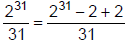So remainder = 2

FERMAT’S THEOREM
If p is a prime number and N is prime to p, then Np -1 - 1 is a multiple of p.

Corollary
Since p is prime, p - 1 is an even number except when p = 2.
Therefore (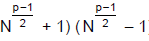) = M(p).

Hence either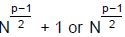-1 is a multiple of p, that is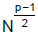= Kp ± 1, where, K is some positive integer.

BASE RULE AND CONVERSION
This system utilizes only two digits namely 0 & 1 i.e. the base of a binary number system is two.
Example: 11012 is a binary number, to find the decimal value of the binary number, powers of 2 are used as weights in a binary system and is as follows:
1 × 23 = 8
1 × 22 = 4
0 × 21 = 0
1 × 20 = 1
Thus, the decimal value of 11012 is 1 × 23 + 1 × 22 + 0 × 21 + 1 × 20 = 13.

CONVERSION FROM DECIMAL TO OTHER BASES
We will study only four types of Base systems,

• Binary system (0, 1)
• Octal system (0, 1, 2, 3, 4, 5, 6, 7).
• Decimal system (0, 1, 2, 3, 4, 5, 6, 7, 8, 9)
• Hexa-decimal system (0, 1, 2, 3, 4, 5, 6, 7, 8, 9, A, B, C D, E, F) where A = 10, B = 11 … ,F= 15.

Let us understand the procedure with the help of an example
Example 1. Convert 35710 to the corresponding binary number.
Solution. To do this conversion, you need to divide repeatedly by 2, keeping track of the remainders as you go. Watch below:
As you can see, after dividing repeatedly by 2, we end up with these remainders: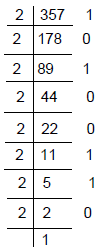These remainders tell us what the binary number is. Read the numbers outside the division block, starting from bottom and wrapping your way around the right-hand side and moving upwards.

Example 2. (357)10 convert to (101100101)2.
This method of conversion will work for converting to any non-decimal base. Just don't forget to include the first digit on the left corner, which is an indicator of the base. You can convert from base-ten (decimal) to any other base.

CONVERSION FROM OTHER BASES TO DECIMAL
We write a number in decimal base as
345 = 300 + 40 + 5 = 3 × 102 + 4 × 101 + 5 × 100

Similarly, when a number is converted from any base to the decimal base then we write the number in that base in the expanded form and the result is the number in decimal form.

Example 1. Convert (1101)2 to decimal base
Solution. (1101)2 = 1 × 23 + 1 x 22 + 0 × 21 + 1 × 20 = 8 + 4 + 1 = 13
So (1101)2 = (13)10

Example 2. Convert the octal no 3456 in to decimal number.
Solution. 3456 = 6 + 5 × 8 + 4 × 82 + 3 × 83
= 6 + 40 + 256 + 1536
= (1838)10

Example 3. Convert (1838)10 to octal.
Solution.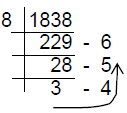= (3456)

Question for Number Systems - Finding Remainders, Number Theory
Try yourself:What is the product of highest 3 digit number & highest 2 digit number of base 3 system?

Example 5. Find the fifth root of (15AA51)19.
Solution. (15AA51)19 = 1.195+ 5.194+ 10.193+ 10.192+ 5.191+ 1.190
= (19+1)5 = 20 (Using binomial theorem)
Therefore, the fifth root is 20

Question for Number Systems - Finding Remainders, Number Theory
Try yourself:In a number system the product of 44 and 11 is 1034. The number 3111 of this system, when converted to the decimal number system, becomes?
Question for Number Systems - Finding Remainders, Number Theory
Try yourself:What is the remainder when (13100 + 17100) is divided by 25?

IMPORTANT POINTS

• The sum of consecutive five whole numbers is always divisible by 5.
• The square of any odd number when divided by 8 will leave 1 as the remainder
• The product of any three consecutive natural numbers is divisible by 6.
• The unit digit of the product of any nine consecutive numbers is always zero.
• For any natural number n, 10n-7 is divisible by 3.
• Any three-digit number having all the digits same will always be divisible by 37.
The document Number Systems - Finding Remainders, Number Theory Notes | Study UPSC Prelims Paper 2 CSAT - Quant, Verbal & Decision Making - UPSC is a part of the UPSC Course UPSC Prelims Paper 2 CSAT - Quant, Verbal & Decision Making.
All you need of UPSC at this link: UPSC

## UPSC Prelims Paper 2 CSAT - Quant, Verbal & Decision Making

67 videos|50 docs|151 tests
 Use Code STAYHOME200 and get INR 200 additional OFF

## UPSC Prelims Paper 2 CSAT - Quant, Verbal & Decision Making

67 videos|50 docs|151 tests

### How to Prepare for UPSC

Read our guide to prepare for UPSC which is created by Toppers & the best Teachers

Track your progress, build streaks, highlight & save important lessons and more!

,

,

,

,

,

,

,

,

,

,

,

,

,

,

,

,

,

,

,

,

,

,

,

,

,

,

,

;